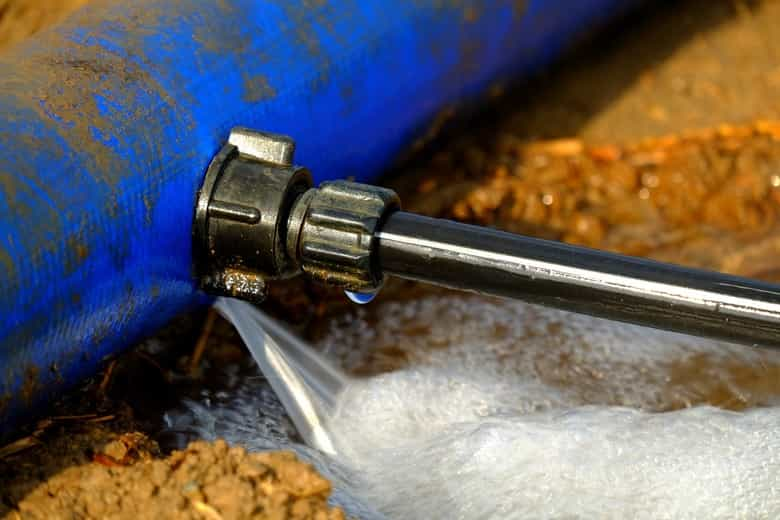# Flow Calculators

## Our best flow calculators

In this category we will find useful tools for the instrumentation engineer, such as the measurement of Normal, Current and Standard flow or the calculation of the amount of product that leaks through a pipe. We will progressively add more tools to this section. The results of all calculators are exportable in spreadsheet format. If you have any questions or require further clarification, do not hesitate to contact us.### Leak Rate Calculator

This gas leak rate calculator or liquid leak rate calculator let you calculate the amount of mass expressed in kilograms of fluid leaked to the atmosphere. The results are exportable in spreadsheet format.### Flow Rate Calculator

Flow is measured as a volume or mass per unit time. This calculator let you calculate the flow rate of any liquid or gas fluid starting from different linear, volumetric flow rate, mass flow rate or normal flow rate.The results are exportable in spreadsheet format.### Hose Flow Rate Calculator

This Flow Rate Calculator will calculate the average flow rate of the fluid based on the hose diameter, pressure and length of the hose.